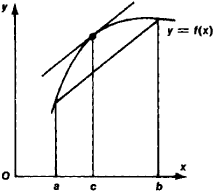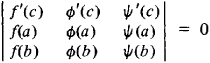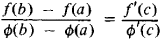# Mean Value Theorem

(redirected from Mean-value theorem)

## mean value theorem

[′mēn ′val·yü ‚thir·əm]
(mathematics)
The proposition that, if a function ƒ (x) is continuous on the closed interval [a,b ] and differentiable on the open interval (a,b), then there exists x0, a <>x0<>b, such that ƒ(b) - ƒ(a) = (b-a)ƒ′(x0). Also known as first law of the mean; Lagrange's formula; law of the mean.
McGraw-Hill Dictionary of Scientific & Technical Terms, 6E, Copyright © 2003 by The McGraw-Hill Companies, Inc.
The following article is from The Great Soviet Encyclopedia (1979). It might be outdated or ideologically biased.

## Mean Value Theorem

one of the fundamental results of the differential calculus relating an increment of a function f(x) and the values of its derivative. In analytic terms,

f(b) — f(a) = (b — a)f’(c)

where c is some number satisfying the inequality a < c < b. Formula (1) is valid if the function f(x) is continuous on the segment [a, b] and has a derivative at each point of the interval (a, b). In geometric terms (see Figure 1), formula (1) states that the tangent to the curve y = f(x) at a suitable point [c, f(c)] is parallel to the chord passing through the points [a, f(a)] and [b, f(b)]. The mean value theorem was discovered by J. Lagrange in 1797.Figure 1

Among the different generalizations of the mean value theorem, note Bonnet’s mean value formulaand its particular case, Cauchy’s mean value formulaReferences in periodicals archive ?
In Section 3 we give a general mean-value theorem for the fuzzy integral, and see its relevance to optimization with inexact constraints.
In this section we give a mean-value theorem for the fuzzy integral.
The next result is a counterpart of the mean-value theorem in the classical measure theory.
does not change its positivity on [m,M], applying the integral mean-value theorem on (19) we get that there exists [xi], [member of] [m, M] such that
does not change its positivity on [m,M], applying the integral mean-value theorem on (43) we get that there exists [xi], [member of] [m, M] such that
In the following two sections of our paper we give some further generalizations of the Jensen-type inequalities on time scales allowing negative weights, and we also give the mean-value theorems of the Lagrange and Cauchy type for the functionals obtained by taking the difference of the left-hand side and right-hand side of these new inequalities.
We subsequently get with the help of the mean-value theorem of Cauchy that
We can write with the help of the mean-value theorem of Lagrange that
Using the mean-value theorem, (3.1) and the estimate on [U.sup.(0)], we conclude that
Using the mean-value theorem and the equation for [Z.sup.(n)], we have
Using the mean-value theorem, from (2.4) and (4.6), conclude that W (P, [tau]) satisfies
Also, equations (A5) and (A6), along with the Mean-Value Theorem, imply (A9) [Mathematical Expression Omitted] for some [q.sub.1] in ([q.sub.11] [sup.*], [q [bar].sub.1]), (A10) [Mathematical Expression Omitted] for some [q.sub.2] in ([q [bar].sub.22], [q.sub.2] [sup.*]).

Site: Follow: Share:
Open / Close